Last Update: Dec 31, 2022

This tutorial is part of a series. You can see the other tutorials below:

Beginning C#
1. Introduction
2. Namespaces
3. Coding Basics
4. Variables and Types
5. Selection Statements
6. Iteration Statements

In part 6 of my learning C# tutorial series we’re going to talk about Iteration Statements in C#. In part 5 we went over C# selection statements, which control program flow with true and false values using statements that execute a statement once based on a criteria. This is great for statements that need to execute in a sequence, but Iteration statements simplify statements that need to be executed in repetition.

### What exactly is an Iteration?

An Iteration is just a fancy word for a loop. An Iteration statement will perform operations a set number of times until you tell it to stop, with a true or false value.

Iteration statements also depend on an expression being evaluated until a that expression evaluates to false. This is called “loop termination criteria” and depends on Boolean values being evaluated.

I’ll explain with some code. Let’s write something that counts ten items procedurally.

``````using System;

namespace tutorials {

public class TestDoWhile
{
public static void Main ()
{
Console.WriteLine("Count is 0");
Console.WriteLine("Count is 1");
Console.WriteLine("Count is 2");
Console.WriteLine("Count is 3");
Console.WriteLine("Count is 4");
Console.WriteLine("Count is 5");
Console.WriteLine("Count is 6");
Console.WriteLine("Count is 7");
Console.WriteLine("Count is 8");
Console.WriteLine("Count is 9");
}
}
}
``````

Note: You might be wondering why we aren’t counting from 1 to 10. That’s because computers start counting at zero, not one and you have to start getting used to that idea if you’re a beginner. There are ten elements here, but since we started at zero the last digit is 9.

While it’s pretty easy to understand what’s going on here you can tell right away there is a need for optimization. Part of being a “lazy programmer” is not wanting to repeat yourself. This adds time and increases the amount of mistakes.

Look how much cleaner and easier this code is:

``````using System;

namespace tutorials {

public class TestDoWhile
{
public static void Main ()
{
int i = 0, limit = 10;
do
{
Console.WriteLine("Count: " + i);
i++;
} while (i < limit);
}
}
}
``````

This code produces the same output yet it’s much less to write and easier to understand. The expression “do” executes and outputs a number (represented by i) then increments that number by adding 1 to it. This do statement would run forever if we let it, so the 2nd part of it is the while statement that evaluates the expression “i < limit”. While checks to see if i is smaller than the limit we specified, and if it is then it returns to execute do again.

Next I’ll explain the Iteration Statements available in C#, and how they work.

Note: Like my other tutorials I will be eliminating the using statements and namespace / class declarations because you shouldn’t repeat yourself in tutorials either ;)

### While statement

The while statement is frequently used in code due to it’s simplicity and speed. In the example above I used a combination of do and while to create a do-while loop, but while can operate on it’s own. Let’s do the count to 10 with just a while loop and see how it works:

``````static void Main()
{
int i = 0, limit = 10;
while (i < limit)
{
Console.WriteLine("Count: " + i);
i++;
}
}
``````

This loop is pretty much as simple as it gets. The while simply runs while the expression evaluates to true .

In plain English:

• While our value (i) is less than another value (limit) execute,
• Write out the value (i)
• Increment the value (i)

This loop will repeat until the expression (i < limit) evaluates to false. If it is already set to false, nothing in the while loop will execute. Type the following in and run it:

``````static void Main()
{
int i = 10, limit = 10;
while (i < limit)
{
Console.WriteLine("Count: " + i);
i++;
}
}
``````

When you run this, you’ll notice nothing happens. This is because (i) is not less than (limit) so the loop never runs. This is ideal for most situations, but you may want your loop to run at least once, which brings us to the next loop.

### Do statement (with while)

I used the do statement in the first example in combination with the while statment. Do executes a statement or block of statements until a specified expression evaluates to FALSE. You can use do without braces if it’s only a single statement:

``````do Console.WriteLine("Count: " + i); while (i < limit);
``````

but if you need to execute more than one statement you’ll need to enclose them in braces:

``````do
{
Console.WriteLine("Count: " + i);
i++;
} while (i < limit);
``````

The mechanics of this should be fairly obvious: in plain English we want to:

• write a value (i) to the console
• increment the value
• repeat while that value is less than another (limit)

This is pretty simple, but take a note of the order of operations with this loop. The thing to remember about a do-while loop such as the one above is this:

A do-while loop will always execute then evaluate an expression to see if it needs to continue looping. The statement will always be executed at least once, while a while loop will only execute if a condition is evaluated as true. This is an important distinction.

We can break out of a while loop early if needed:

``````int i = 0, limit = 10;
do
{
if (i == 7)
{
break;
}else
{
Console.WriteLine("Count: " + i);
}
i++;
}
while (i < limit);
``````

When you run this you will see the following output:

Count: 0 Count: 1 Count: 2 Count: 3 Count: 4 Count: 5 Count: 6

This is because you put in a condition that evaluated whether i was 7 and if so, terminate the loop. This can come in pretty handy. You can also use goto, return and flow to break out of this loop as well. There are times where you may want to check for something in each iteration and if it happens, break out of it to correct it or stop the process.

### For Statement

The for loop is a little more tricky to understand at first, but it performs the same function as a while loop. The for loop isn’t faster or any more powerful, but it is easier to construct and read so it’s mostly a programmer advantage (very important).

Here’s an example of a for loop that counts to 10:

``````public static void Main ()
{
for (int i = 0; i < 10; i++)
{
Console.WriteLine(i);
}
}
``````

When you compile and run this it should look pretty familiar to you. The output is the same, but as you can see constructing the loop is easier and there is less code to look at. Let’s explain how it works in plain English:

• test to see if our initial value (i) is less than ten
• if so, increment it and execute next expression
• write out the value (i)

This is my preferred method of looping most of the time because it’s so easy to set up. Here is how the function works: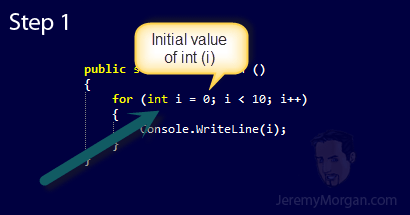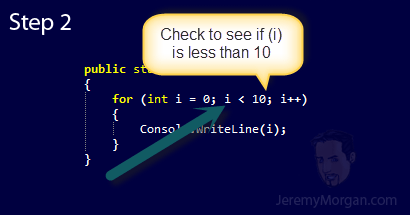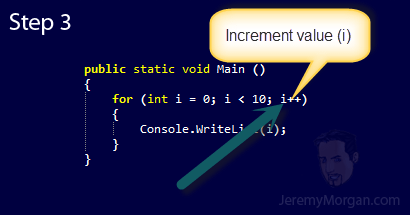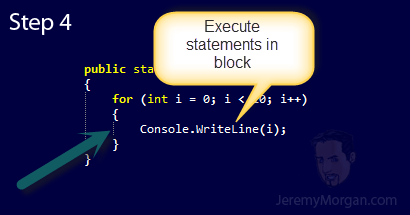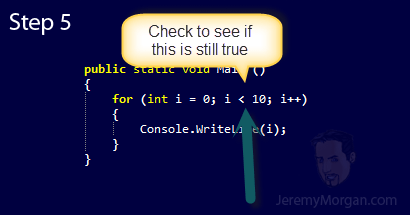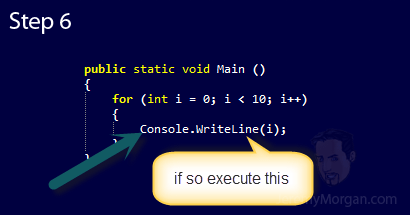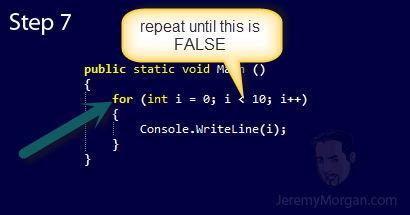I hope this has helped clear up how the for loop works. This is a really quick way to iterate through a finite number fairly quick.

### Foreach statement

The foreach statement is a little different than for in that it repeats a group of statements for each element in an array, object or collection. It is used for reading those elements in the sequence defined by the foreach statement.

Here is an example of our count to 10 app with a foreach statement:

`````` public static void Main ()
{
int[] ourArray = new int[] { 0, 1, 2, 3, 4, 5, 6, 7, 8 , 9 };

foreach (int i in ourArray)
{
System.Console.WriteLine(i);
}
}
``````

As you can see in this example it’s a little different because we’re creating an array on line 3 that we iterate though. Since this is an integer array we create the int(i) to represent the element of the array. Then we execute everything in the brackets each time a new element in the array is found. While there are new elements in i we will display them, then as soon as there are no more we exit from the loop.

But this doesn’t just work on integer arrays, it works on all types of arrays and objects where you use an iterator. Here’s an example with strings:

``````public static void Main ()
{
string[] ourArray = new string[] { "C# Tutorials", "Python Tutorials", "Underwater Basket Weaving" };

foreach (string s in ourArray)
{
System.Console.WriteLine(s);
}
}
``````

Notice how the variable (s) is pulled from the array and displayed until there are no more in the array. This is a great way to iterate through arrays, lists, collections and objects.

Note that you should generally only use this for read only operations. If you start messing with items of an array as it’s being traversed you get some pretty unexpected results.

The foreach statement is great for working with data objects, and is extremely powerful and easy to use.

### Conclusion

In this tutorial we explored four kinds of iterations in C#:

• while
• do
• for
• foreach

These are commonly used core parts of C# development, but it’s far from the only way to iterate data in C#. In many ways LINQ is taking over this area pretty well, and I’ll cover that in future issues. But learning these statements well will help you on your way to becoming a better C# Programmer.

### Can you beat my SkillIQ Score in C#??I think you can! Take the C# test here to see where you stand!Published: Feb 1, 2013 by Jeremy Morgan. Contact me before republishing this content.